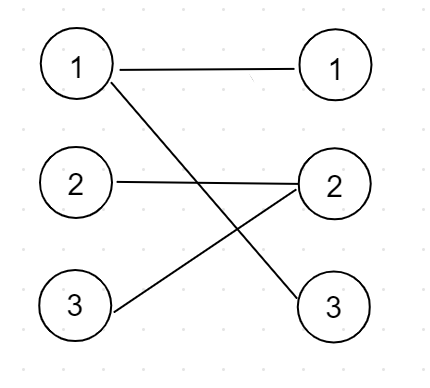## POJ 3041 Asteroids（二分图最大匹配）

###题目链接###1、对于每条边，只要有其中一个端点被选取，则该条边所代表的行星就可以被摧毁。同样，如果选取了这个端点，则所有与这个端点连接的所有行星都会被一次摧毁。

2、对于样例，假设我选取了 1（左集合）--- 1（右集合） 这条边，则说明我已经选择了 横着切第一行 或者 竖着切第一列 。那么与 1（左集合） 所连接的所有边代表的小行星都会被消除，对于 1（右集合） 同理。

```#include<iostream>
#include<algorithm>
#include<string.h>
#define maxn 1008
using namespace std;
int n,m,e,cnt;
int cx[maxn],cy[maxn];
bool vis[maxn];
struct Edge
{
int to;
int next;
}edge[maxn*maxn];
{
edge[++cnt].to=v;
return;
}
inline int dfs(int u)
{
int v=edge[i].to;
if(!vis[v]){
vis[v]=true;
if(cy[v]==0||dfs(cy[v])){
cx[u]=v;
cy[v]=u;
return 1;
}
}
}
return 0;
}
int main()
{
// =freopen("testdata (7).in","r",stdin);
scanf("%d%d",&n,&m);
int A,B;
int ans = 0;
for(int i=1;i<=n;i++){
if(!cx[i]) {memset(vis,0,sizeof(vis)); ans += dfs(i);}
}
printf("%d\n",ans );
}```